# 【评分卡】评分卡入门与创建原则——分箱、WOE、IV、分值分配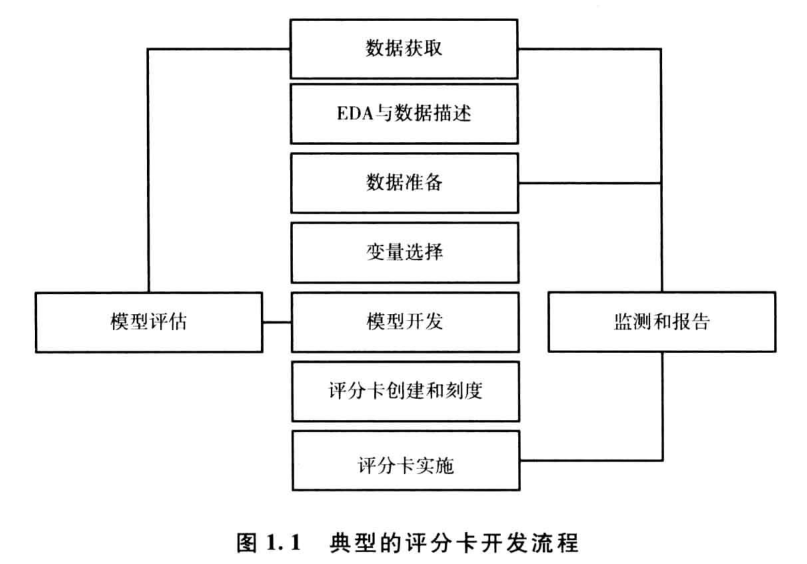## 变量分析

### WOE

WOE(weight of Evidence)字面意思证据权重，对分箱后的每组进行。假设good为好客户（未违约），bad为坏客户（违约）。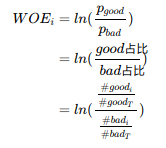#good(i)表示每组中标签为good的数量，#good(T)为good的总数量；bad相同。

### IV

IV(information value)衡量的是某一个变量的信息量，公式如下：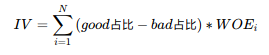N为分组的组数；
IV可用来表示一个变量的预测能力。

IV 预测能力
<0.03 无预测能力
0.03~0.09
0.1~0.29
0.3~0.49
>=0.5 极高

1. 组间差异大
2. 组内差异小
3. 每组占比不低于5%
4. 必须有好、坏两种分类

### 举例说明

Age good bad WOE
<18 50 40 $ln(\frac{50/330}{40/220}) = -0.182321556793955$
18~30 100 60 $ln(\frac{100/330}{60/220}) = 0.105360515657826$
30~60 100 80 $ln(\frac{100/330}{80/220}) = -0.182321556793955$
>60 80 40 $ln(\frac{80/330}{40/220}) = 0.287682072451781$
ALL 330 220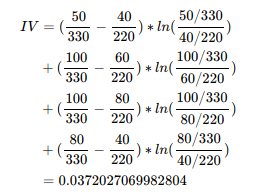## 建立模型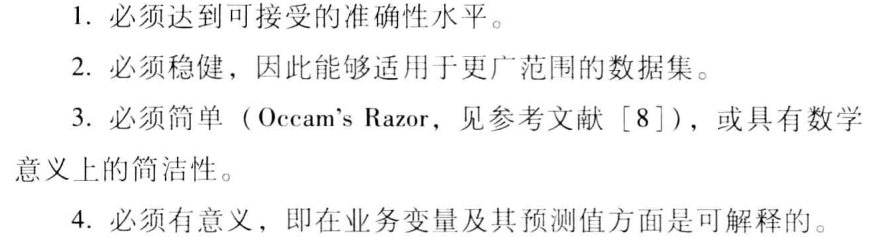## 评分卡

### 评分卡计算方法

odds为good用户概率（p）与bad用户概率（1-p）的比值。
$odds=\frac{坏客户概率}{好客户概率}=\frac{p}{1-p}$

$score_总=A+B*ln(odds)$

$\begin{cases} P_0 &= A+Bln(\theta_0) \\ P_0+PDO &= A+Bln(2\theta_0) \end{cases}$

$\begin{cases} B &= \frac{PDO}{ln2} \\ A &= P_0-Bln(\theta_0) \end{cases}$

$P_0$$PDO$ 的值都是已知常数，可以设置$P_0 = 20$$PDO = 600$

### 分值分配

$p = \frac{1}{1+e^{-\theta^Tx}}$

$ln(\frac{p}{1-p})=\theta^Tx$

$ln(\frac{p}{1-p})=ln(odds)$

$ln(odds)=\theta^Tx =w_0+w_1x_1+···+w_nx_n$

$score_总 = A+B*(\theta^Tx)=A+B*(w_0+w_1x_1+···+w_nx_n)$
$=(A+B*w_0)+B*w_1x_1+···+B*w_nx_n$

$(A+B*w_0)$为基础分数，$B*w_1x_1,···,B*w_nx_n$为每个变量对应分配到的分数。

$x_1$ 1
2

$i$
$(B*w_1)*WOE_{11}$
$(B*w_1)*WOE_{12}$
···
$(B*w_1)*WOE_{1i}$
$x_2$ 1
2

$j$
$(B*w_2)*WOE_{21}$
$(B*w_2)*WOE_{22}$
···
$(B*w_2)*WOE_{2j}$
··· ··· ···
$x_n$ 1
2

$k$
$(B*w_n)*WOE_{n1}$
$(B*w_n)*WOE_{n2}$
···
$(B*w_n)*WOE_{nk}$

《信用风险评分卡研究》Mamdouh Refaat著
《互联网金融时代消费信贷评分建模与应用》单良著

《统计学习方法》李航著